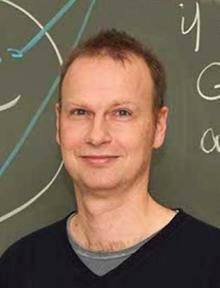## Symmetry and Similarity

• 27 November 2019
4:30 PM
• Governor's Palace, Moravské náměstí 1a, Baroque HallMartin Grohe is a German mathematician and computer scientist. His research interest is in theoretical computer science interpreted broadly, including logic, algorithms and complexity, graph theory, theoretical aspects of machine learning, and database theory.

He is a Professor for Theoretical Computer Science at the RWTH Aachen, where he holds the Chair in Logic and the Theory of Discrete Systems. He received his PhD in Mathematics at Freiburg University in 1994 and then spent a year as a visiting scholar at Stanford and the University of California at Santa Cruz. Before joining the Department of Computer Science of RWTH Aachen in 2012, he held positions at the University of Illinois at Chicago, the University of Edinburgh, and the Humboldt University at Berlin.

Grohe won the Heinz Maier–Leibnitz Prize awarded by the German Research Foundation in 1999. He was elected as an ACM Fellow in 2018 for "contributions to logic in computer science, database theory, algorithms, and computational complexity".

#### Abstract

Symmetry is a fundamental concept in mathematics, the sciences, and beyond.

Understanding symmetries is often crucial for understanding structures. In computer science, we are mainly interested in the symmetries of combinatorial structures. Computing the symmetries of such a structure is essentially the same as deciding whether two structures are the same ("isomorphic"). Algorithmically, this is a difficult task that has received a lot of attention since the early days of computing. It is a major open problem in theoretical computer science to determine the precise computational complexity of this "Graph Isomorphism Problem".

One of the earliest applications of isomorphism testing was in chemistry, more precisely chemical information systems. Today, applications of isomorphism testing and symmetry detection are ubiquitous in computing.
Prominent examples appear in optimisation, malware detection, and machine learning. However, in many of these applications, we only need to decide if two structures are sufficiently similar, rather than exactly the same. It turns out that determining how similar two structures are is an even harder computational problem than deciding whether they are isomorphic.

My talk will be an introduction to algorithmic aspects of symmetry and similarity, ranging from the fundamental complexity theoretic "Graph Isomorphism Problem" to applications in optimisation and machine learning.

#### Where?

Governor's Palace, Moravské náměstí 1a, Baroque Hall